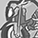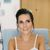# QlikView App Dev

Discussion Board for collaboration related to QlikView App Development.

Announcements
Join this live chat April 6, 10AM EST - QlikView to Qlik Sense REGISTER
cancel
Showing results for
Did you mean:Specialist

## What does "'#.#%'" means ??

In the following example:

=num(Sum({<Year={2011}>} Margin)/Sum({<Year= {2011}>} Sales), '#.#%')

What does "'#.#%'" means ??  ANd from where it comes from ?? Do we have to select some property to show this text ??

1 Solution

Accepted SolutionsSpecialist
 nikhil garg wrote: Hey, But the expression where it is used if i remove"#.#%" then answer is :  .88675..... And when i use "#.#" , it shows 88.7%. It means , it not only multiply by 100 but also doing rounding off. Am i right ??

Hello nikhil,

You are correct that the formatting string will show a display value that has been rounded off, but all mathematical calculations on the field will continue to calculate against the original value.  You're showing it rounded off but your changes haven't really been applied, so to speak.  All data in QlikView that has a display format is essentially Dual() data type, a combination of a display value with a numeric value.

With the formatting string in this case, even though you see 88.7, the underlying value will still hold .88675.  88.7 is the display value, .88675 is the numeric value.

If you truly want to round the number to a 10th of a percent, apply the Round() function, and then the display value can be assigned to match the calculation value.

In the event you want to force the display of a digit in a formatting string, use the number zero in place of #.

'#.00 %'  //will always show percent to two decimal places even if zero

'#.## %'  //will only show two decimal places when there are two significant digits

9 RepliesMVP

Hi,

#.# set you number to display upto digit.

Like if your

Final output is 24.1222222 then #.# return all digits.

If it modify like #.00 then it will show you 24.12

It is number format setting.

For detail you can see num() in Help menu.

Regards

Great dreamer's dreams never fulfilled, they are always transcended.Creator III

this belong to the num function.

its how do you want to show the number (the num format) of  the expresion-Sum({<Year={2011}>} Margin)/Sum({<Year= {2011}>} Sales),

"#.#%"->number.number and %MVP

Hi,

It formats the given number into percentage and shows one decimal point.  For example

=Num(1, '#.#%') - returns 100%

=Num(1.5989, '#.#%') - returns 159.9%

Hope this helps you.

Regards,

Jagan.Specialist
Author

Hey,

But the expression where it is used if i remove"#.#%" then answer is :  .88675.....

And when i use "#.#" , it shows 88.7%. It means , it not only multiply by 100 but also doing rounding off. Am i right ??MVP

Hi Nikhil,

When you use % symbol it will multiply the number with 100 (to convert to %).

Regards,

Jagan.Specialist
Author

Hey,

Thanx but what is think is no of times "#" is placed after "#." will show that number of digits. And if "%" is placed , it will simply multiply by 100 and show it in %.MVP

Hi Nikhil,

If you are giving 5 #s after . then it will show 5 decimal numbers after 0.

Normally 1 in percentage is 100%.

Hope you understand.

Regards,

Jagan.Specialist
 nikhil garg wrote: Hey, But the expression where it is used if i remove"#.#%" then answer is :  .88675..... And when i use "#.#" , it shows 88.7%. It means , it not only multiply by 100 but also doing rounding off. Am i right ??

Hello nikhil,

You are correct that the formatting string will show a display value that has been rounded off, but all mathematical calculations on the field will continue to calculate against the original value.  You're showing it rounded off but your changes haven't really been applied, so to speak.  All data in QlikView that has a display format is essentially Dual() data type, a combination of a display value with a numeric value.

With the formatting string in this case, even though you see 88.7, the underlying value will still hold .88675.  88.7 is the display value, .88675 is the numeric value.

If you truly want to round the number to a 10th of a percent, apply the Round() function, and then the display value can be assigned to match the calculation value.

In the event you want to force the display of a digit in a formatting string, use the number zero in place of #.

'#.00 %'  //will always show percent to two decimal places even if zero

'#.## %'  //will only show two decimal places when there are two significant digitsSpecialist
Author

Hey,

THanx a lot